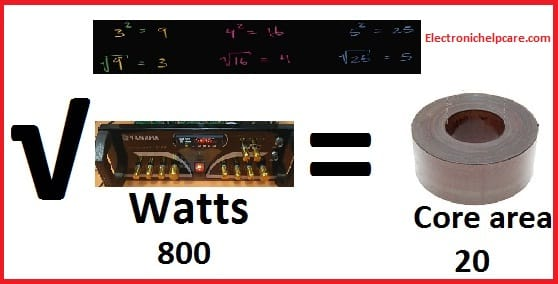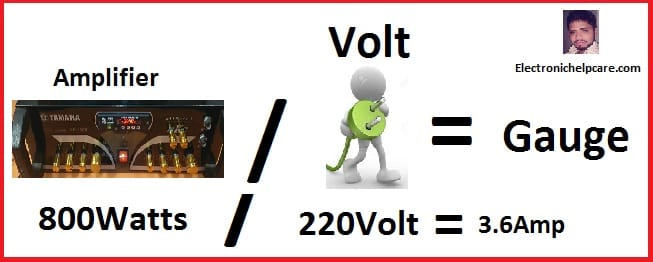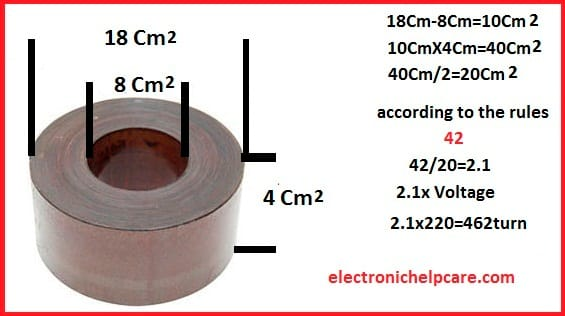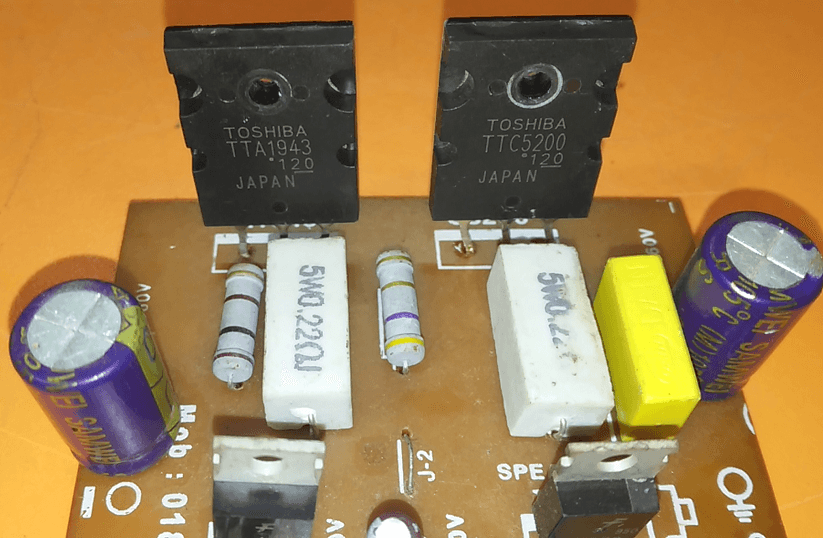Breaking News

# how to make toroidal transformer core

## how to make toroidal transformer core:

How to make Toroidal transformer core: Here in this Video you can learn how to make the toroidal transformer.

First, you have to take decisions that how many watts transformer you need. Take core for the transformer according to watts.if our core area is 20. needs the ampers is 800 Watts. now we need to calculate. which wire we need to use?

Gauge………….=………………Ampere

7……………………………………44.2

8……………………………………33.3

9……………………………………26.5

10………………………………….21.2

11………………………………….16.6

12………………………………….13.5

13………………………………….10.5

14………………………………….8.3

15………………………………….6.6

16………………………………….5.2

17………………………………….4.1

## 18………………………………….3.2

19………………………………….2.6

20………………………………….2.0

21………………………………….1.6

22………………………………….1.2

23………………………………….1.0

24………………………………….0.8

25………………………………….0.6

26………………………………….0.5

27………………………………….0.4

28………………………………….0.3

29………………………………….0.29

30………………………………….0.22

## How do you know that which Gauge you need?Calculating the gauge according to our amplifier Watts. Our amplifier Watts is 800. And the input voltage is 110. So 800/110=7.27Amp wire need . Or our input voltage is 220. So 8oo/220=3.36amp wire has to use.

## Now you need to calculate the Winding turn. How many turns do you need?18cm2-8cm2=10cm2

10cm2x4cm2=40cm2

40cm2/2=20cm2 is the core area.

According to the calculation rules, 42 is the key to find out the turns per voltage

So 42/20cm2=2.1 turn per voltage.

as your input voltage. if 110volt.

110×2.1=231turn need.

Or for 220×2.1=484turn need.

## Calculation the sec wire turn and Gauge:

According to the Ampere. how many amperes do you need?

if you need 20 Ampere you need to use 10 number gauge.

Always think where you use the transformer. here we are using this transformer to an amplifier

so we must think how many amperes need to run this amplifier. here this amplifier used transistor 5200 and 1943.here you can see the transistor. this transistor needs 1.2 Ampere to run. So if you use 8 transistors. 8×1.2=9.6 Ampere needs to make this amplifier. the Ampere is depended on your amplifier.

now calculate the sec volt.

we need 35-0-35 volt

so 2.1×35=73.5 Turn need. we give 74 Turn in Double.

we give double because the transformer is a center tub transformer.

## Lets Watch the video How to make the transformer?

transistor circuit diagram of 2sa1943 and 2sc5200

Thanks a lot to be with us. If you want another post then please visit our website.

We have another post for you. like as repairing amplifier,

Please like comment and share our post.Ramen Bairagee

## Step-down Transformer

How to make Step-down Transformer: At first, we need to know how to make transformer? To …

### One comment

1.Thanks I would like to know more about coil transformers